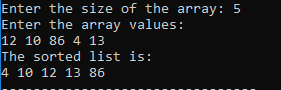# Selection Sort in C++: The Complete Guide

Like Bubble Sort, Selection Sort is a sorting algorithm, especially an in-place comparison sort. The selection sort algorithm is based on finding the min or max element or item in the unsorted array and then putting it in its correct position in the sorted array.

## Selection Sort in C++

Selection sort in C++ is a straightforward sorting algorithm. A selection sorting algorithm is an in-place comparison-based algorithm in which the list or an array is divided into two parts, the sorted part at the leftmost end and the unsorted part at the rightmost end. So, initially, the sorted part is empty, and the unsorted part is an entire list.

The smallest element is selected from an unsorted array and swapped with the leftmost element, and that element becomes part of a sorted array. This process continues moving the unsorted array boundary by the component to the right. Finally, we get the sorted values. In conclusion,

The selection sort algorithm divides an array into two parts:

1. A sorted subarray
2. The unsorted sub-array, from where we have to pick the smallest element and then put it into the sorted array.

## Algorithm of Selection Sort:

Selection(A,N)

Step1: Repeat step 2 and 3 for (k=0;k<n-1;k++)

Step2: Call MINIMUM(A,K,N,LOC)

Step3: Swap A[K] and A[LOC]

Step4: Exit

## MINIMUM(A,K,N,LOC)

Step1: Set min=A[K] and LOC=K

Step2: Repeat step 3 and 4 for(i=k;i<n;i++)

Step3: if min>a[i]

Step4: set min=a[i] and LOC=i

Step5: Return LOC

## Explanation with an example

From the algorithm, we can understand that, as said above, the array is in two parts, one part in sorted order and another in unsorted order. So, we find the smallest value from that unsorted order and put it into a sorted order list.

For example, Let’s take a list of integers:

 Array Number 12 10 86 4 13 Location 0 1 2 3 4

## First Pass:

When K=0; SELECTION() will call MINIMUM() and check the smallest value from the list, so, here in the first pass, the lowest value will be 4, and the MINIMUM() will return the location of 4, that is LOC=3.

Then, SELECTION() will swap the value with current A=12. So, after swapping the value new array will be:

 Array Number 4 10 86 12 13 Location 0 1 2 3 4

## Second Pass:

Now, K=1 and again SELECTION() will call MINIMUM(), and then it will check the smallest value from the unsorted array, 10, and then it will return the location of 10, i.e., LOC=1.

The final array will be the same as 10 is at its correct position.

## Third Pass:

Now, K=2 and again SELECTION() will call MINIMUM(), and then it will check the smallest value from the unsorted array, that is 12, and then it will return the location of 12, i.e., LOC=3.

Then, SELECTION() will swap the value with current A=86. So, after swapping the value new array will be:

 Array Number 4 10 12 86 13 Location 0 1 2 3 4

## Fourth Pass:

Now, K=3, and again SELECTION() will call MINIMUM(), and then it will check the smallest value from the unsorted array, that is 13, and then it will return the location of 13, i.e., LOC=4.

Then, SELECTION() will swap the value with current A=86. So, after swapping the value new array will be:

 Array Number 4 10 12 13 86 Location 0 1 2 3 4

## Selection Sort Pseudocode

procedure selection sort
list  : array of items
n     : size of list

for i = 1 to n - 1
/* set current element as minimum*/
min = i

/* check the element to be minimum */

for j = i+1 to n
if list[j] < list[min] then
min = j;
end if
end for

/* swap the minimum element with the current element*/
if indexMin != i  then
swap list[min] and list[i]
end if
end for

end procedure

## C++ Program of Selection Sort

See the below code of the selection.cpp.

#include<bits/stdc++.h>
using namespace std;

int minimum(int a[],int n, int k, int loc)
{
loc=k;
int min=a[k];
for(int i=k;i<n;i++)
{
if(a[i]<min)
{
min=a[i];
loc=i;
}
}
return loc;
}
int selection(int a[],int n)
{
int loc=-1,k;
for(k=0;k<n-1;k++)
{
int loc1=minimum(a,n,k,loc);
int temp=a[k];
a[k]=a[loc1];
a[loc1]=temp;
}
cout<<"The sorted list is:\n";
for(k=0;k<n;k++)
cout<<a[k]<<" ";
}
int main()
{
int n;
cout<<"Enter the size of the array: ";
cin>>n;
int a[n],i;
cout<<"Enter the array values:\n";
for(i=0;i<n;i++)
{
cin>>a[i];
}
//calling selection function
selection(a,n);
return 0;
}

### Output:## Time Complexity:

If we want to find the minimum element from the array of N elements, N−1 comparisons are required. After putting the minimum item in its proper position, the size of an unsorted array reduces to N−1, and then N−2 comparisons are necessary to find the minimum in the unsorted array.

Therefore (N−1) + (N−2) + ……. + 1 = (N⋅(N−1))/2 comparisons and N swaps result in the overall complexity of O(N2).

So, the time complexity for selection sort is O(n2) as there are two nested loops.

## Auxiliary Space: O(1)

The good thing about selection sort is that it never makes more than O(n) swaps and can be helpful when memory writing is a costly operation.

That’s it for this tutorial.

Categories C++

### 2 thoughts on “Selection Sort in C++: The Complete Guide”

1.This Post explains everything is detail about the selection sort algorithm.. Thanks alot dear. great work..!

2.